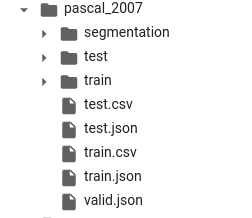# Capter 6 - File not found in training, but file is in validation dir

Hello Fastai crew!

First post here…
Context - notebook 06 - multicat

Created a dataset consisting of one csv, plus train and validate folders with their respective pictures.

The csv file I created has the same format as in the exercise. However, when I train via `learn.fine_tune()`, the error message `FileNotFoundError: [Errno 2] No such file or directory: '/content/gdrive/MyDrive/dest/dataset11/**train**/a-020_label_85.jpg`
However, this file is in reality in the validation set.

`dblock = DataBlock(blocks=(ImageBlock, MultiCategoryBlock),`
` splitter=splitter,`
` get_x=get_x,`
` get_y=get_y,`
`item_tfms = RandomResizedCrop(128, min_scale=0.35))`

`dls = dblock.dataloaders(df)`

And the other relevant functions:
`def get_x( r ): return path/'train'/r['fname']`
`def get_y( r ): return r['labels'].split(' ')`

```````def splitter(df):`
`train = df.index[df['is_valid'] == 0].tolist()`
`valid = df.index[df['is_valid'] == 1].tolist()`
`return train,valid`
``````

Can you share some insight as to what might be causing the confusion between training and validation sets?

Update:
In the muticat notebook, for the PASCAL_2007 dataset, the folders are structured like so:And for setting up the DataBlock, we used everything in the `train/` folder and split the data set based on the accompanying `train.csv` file’s `is_valid` column. In your case though, you have two separate folders for `train` and `valid`. Therefore `get_x()` has to be updated somehow to return the full path, including the subdirectory path. Maybe you can prepend to `fname` in `train.csv`, the parent directory with `awk` or `apply` and try my previous answer.

Maybe something like this

``````p = lambda x: path/'valid/' if x else path/'train/'
df['fname_full'] = df['is_valid'].apply(p)/df['fname']
``````

Now the column `fname_full` has the full path to the file.

I’m not sure, but with what you have provided, it looks like you want to return `return r['fname']` in `get_x(r)` instead.
Thanks @thatgeeman, that was it… I thought there were `train/ valid/ test/` directories, rather than just `train/ test/`.
a) leave my df as is fine, and copy the iimage files from `valid/` into `train/` and use the original code, or